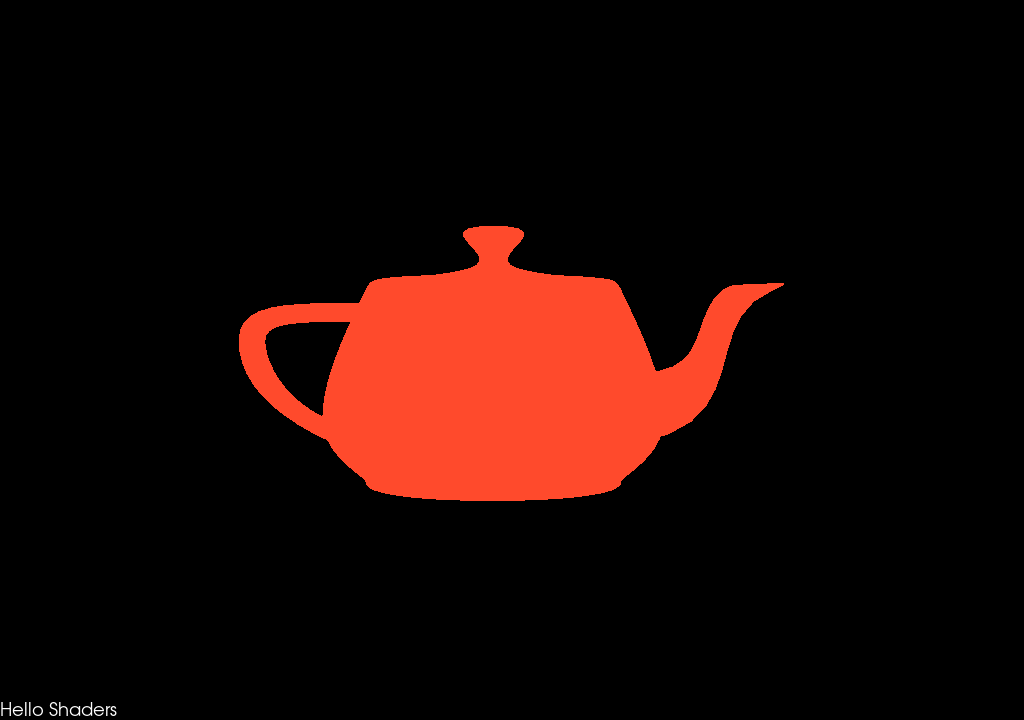# Varying Color#

This example shows how to use shaders to generate a shaded output. We will demonstrate how to load polydata then use a custom shader calls to render a custom shaded model. First, a bunch of imports.

```from fury import window, ui, io, utils
```

```from fury.data.fetcher import fetch_viz_models, read_viz_models
fetch_viz_models()
```
```Dataset is already in place. If you want to fetch it again please first remove the folder /Users/skoudoro/.fury/models
```

Let’s start by loading the polydata of choice. For this example we use the standard utah teapot model. currently supported formats include OBJ, VTK, FIB, PLY, STL and XML

```utah = io.load_polydata(model)
utah = utils.get_polymapper_from_polydata(utah)
utah = utils.get_actor_from_polymapper(utah)
mapper = utah.GetMapper()
```

```vertex_shader_code_decl = \
"""
out vec4 myVertexVC;
"""

"""
myVertexVC = vertexMC;
"""

"""
uniform float time;
varying vec4 myVertexVC;
"""

"""
vec2 iResolution = vec2(1024,720);
vec2 uv = myVertexVC.xy/iResolution;
vec3 col = 0.5 + 0.5 * cos((time/30) + uv.xyx + vec3(0, 2, 4));
fragOutput0 = vec4(col, fragOutput0.a);
"""

block="light")
```

Let’s create a scene.

```scene = window.Scene()

global timer
timer = 0
```

The timer will call this user defined callback every 30 milliseconds.

```def timer_callback(obj, event):
global timer
timer += 1.0
showm.render()
scene.azimuth(5)
```

The shader callback will update the color of our utah pot via the update of the timer variable.

```def shader_callback(_caller, _event, calldata=None):
program = calldata
global timer
if program is not None:
try:
program.SetUniformf("time", timer)
except ValueError:
pass

```
```1
```

Let’s add a textblock to the scene with a custom message

```tb = ui.TextBlock2D()
```

Show Manager

Now that all the elements have been initialised, we add them to the show manager.

```current_size = (1024, 720)
showm = window.ShowManager(scene, size=current_size, reset_camera=False)